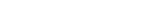MathExamle.com# Addition and Subtraction of fractions

Create worksheets of mathematical examples with addition and subtraction of fractions, select type of a generator and viewing some mathematical examples

### Numerator

Creates examples with addition and subtraction of fractions, the answers are always positive

3 Variants 3 Levels
 1 + 2 9 = 11 22
 5 3 – 4 4 = 5 15
Variant: 1 Level: Normal

### Denominator

Creates examples with addition and subtraction of fractions, the answers can be negative

3 Variants 3 Levels
 – 52 + 5 = 63 7
 1 + 7 = 2 10
Variant: 1 Level: Normal